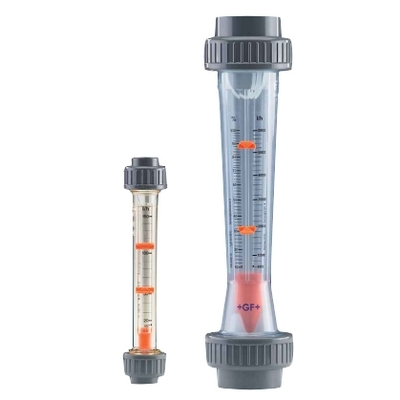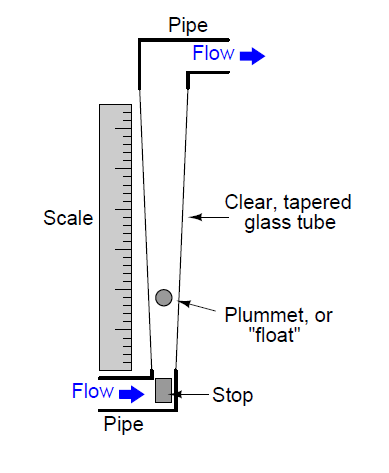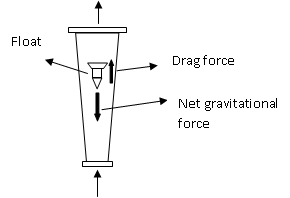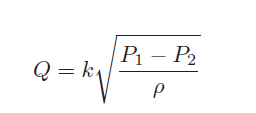# Working of Rotameter / Variable area flowmeter

## What is a rotameter?

Rotameter is variable area flowmeter used to measure fluid flow. It works on the principle of upthrust force exerted by fluid and force of gravity. The buoyant force exerted on an immersed object is equal to the weight of liquid displaced by the object. Under this principle, the rotameter works with float-tapered tube system.It is one of the most commonly used rotameter in the world.

## Construction:A rotameter is made up of a tapered tube and a float inside it. The glass tapered tube has a scale on the surface or a scale is placed adjacent to it, according to purpose.

Tapered tube:

The tapered tube is placed vertically in the flow channel with a conical shape inside. The quantity measured is defined by the height of float going up. Glass tubes are used for both liquid and gas measurement. Metallic tubes are used where the process fluid with high temperature and pressure.

Float:

Stainless steal floats are commonly used, there are different types of metals from lead to aluminium used as floats. A float material, shapes are also varied according to applications considering density.

Spherical shape floats are used for small flows.

## Working:Fluid enters from the bottom of the tapered tube, then some of the fluid strikes directly into the float bottom and others pass aside the float. Now the float experience two forces in opposite direction, darg force upward and gravitational force downward.

Fluid flow moves the float upward against gravity. At some point, the flowing area reaches a point where the pressure-induced force on the floating body exactly matches the weight of the float. The float will find equilibrium when the area around float generates enough drag equal to weight - buoyancy.

As the float weight and gravity are constant, the distance float displaced upward is proportional to the flow velocity of the fluid passing through the tapered tube.

Measuring Principles of Variable Area Flowmeters:However, the difference in this application is that the value inside the radicand is constant since the pressure difference will remain constant and the fluid density will likely remain constant as well. Thus, k will change in proportion to Q. The only variable within k relevant to float position is the flowing area between the float and the tube walls.

• No external power needed

• Simple Reliable Design

• Can Measure Liquid or Gas Flows

• Scale is approximately linear

• It can measure flow rates of corrosive fluid

• Better rangeability

• Low cost and low pressure drop